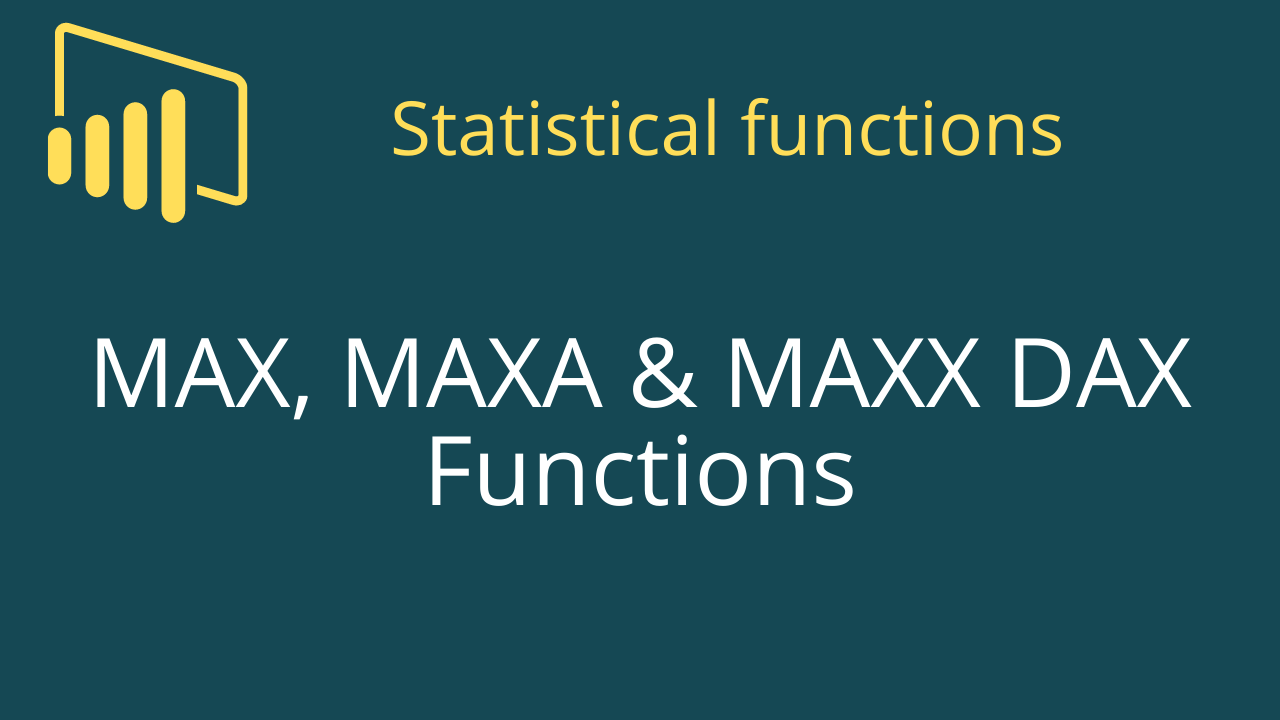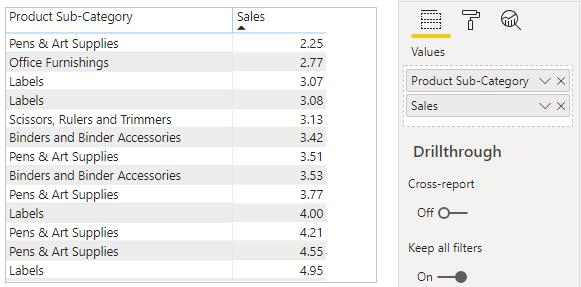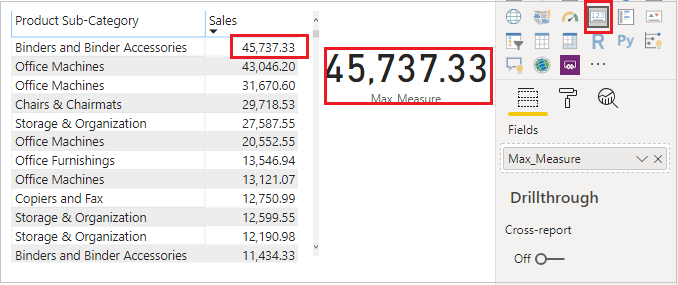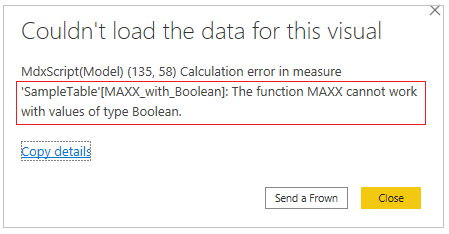Home » DAX » DAX – MAX, MAXA & MAXX Functions

# DAX – MAX, MAXA & MAXX FunctionsPower BI DAX functions MAX, MAXA & MAXX are aggregation functions and returns the largest value in a column. All functions comes under Aggregation functions Dax categories.

### 1- MAX DAX Function:

Returns the largest value in a column.

#### Syntax:

`MAX(<Column>)`

#### Description:

 S no. Parameter Description 1 Column The column in which you want to find the largest numeric value.

#### Let’s understand with an example:

Step 1: Sample Dataset with table visual as below.Aggregation Sample Data Set

Step 2: Now Create Measure  to find maximum sale value from sale column.

Right click on Dataset and click to New measure, then write below DAX

```Max_Measure = MAX(Orders[Sales])

```

Step 3: Now take Card visual from Visualization pane to Power Bi Page & drag measure over it.### Important Points about MAX DAX function:

MAX function support Date, Number, Text data type.

```MAX with Number data type column:-
Max_With_Number = MAX(Orders[Sales])

MAX with Date data type column:-
Max_With_Date = MAX(Orders[Order Date])

MAX with Text data type column:-
Max_With_Text = MAX(Orders[State or Province])
For Text Data type column it will return MAX Alphabet Text.

```

#### It does not support logical value.

```Max_with_Boolean = MAX(SampleTable[Boolean])

Here Boolean column data type is Bit```Boolean

#### You can compare two measures value using MAX function.

```TotalProfit = Sum(Orders[Profit]) ---- 50000

TotalSales= Sum(Orders[Sales])-------10000

Compare_Two_Expression =
MAX([TotalSales],[TotalProfit]) -------50000```

### 2- MAXA DAX Function:

Returns the largest value in a column.

#### Syntax:

`MAXA(<Column>)`

#### Description

 S no. Parameter Description 1 Column The column in which you want to find the largest numeric value.

### Important Points about MAXA DAX function:

MAXA function support Date, Number & Boolean data type.

```MAXA with Number data type column:-
MAXA_With_Number = MAXA(Orders[Sales])

MAXA with Date data type column:-
MAXA_With_Date = MAXA(Orders[Order Date])

MAXA_with_Boolean = MAXA(SampleTable[Boolean])
For Boolean/ Logical value, It will consider True=1, False=0

```

#### It does not support Text data type, It will return 0.

```MAXA with Text data type column:-

MAXA_With_Text = MAXA(Orders[State or Province])

Output=0```

### 3- MAXX DAX Function:

Evaluates an expression for each row of a table and returns the largest value.

#### Syntax:

`MAXX(tablename, expression)`

#### Description

 S no. Parameter Description 1 table The table containing the rows for which the expression will be evaluated. 2 expression The expression to be evaluated for each row of the table.

### Important Points about MAXX DAX function:

MAXX function support Date, Number & Text data type.

```Maxx_With_Number = MAXX(Orders, Orders[Sales])

MAXX with Date data type column:-
Maxx_With_Date = MAXX(Orders,Orders[Order Date])

MAX with Text data type column:-
Maxx_With_Text = MAXX(Orders, Orders[State or Province])

For Text Data type column it will return MAX Alphabet Text.

```

#### It does not support logical value.

```Maxx_with_Boolean = MAXX(SampleTable, SampleTable[Boolean])

Here Boolean column data type is Bit```MAXX Dax

#### You can use Filter condition with MAXX Dax function

Suppose you want to get MAX sales only from “Furniture” category, so you can use Filter DAX with MAXX.

```MAXX_With_Filter =

MAXX(

FILTER(Orders, Orders[Product Category]="Furniture"),

Orders[Sales]

)```

### Watch complete practical video:

Hope you enjoyed the post. Your valuable feedback, question, or comments about this post are always welcome or you can leave us message on our , we will revert to you asap.

## 1 thought on “DAX – MAX, MAXA & MAXX Functions”

1.This is a baby question but it’s got me stumped!

Working in Power BI Desktop I want to find the maximum value of a variable … I used the MAX() function in the way that you have described here and this is what I have found:

Max Sales = MAX(Orders[Sales])
Max Sales2 =MAX([Sales])

Both work and they give me the same answers when I create a table with Customer Segment and these two measures.

In my own file of completely different values and variables